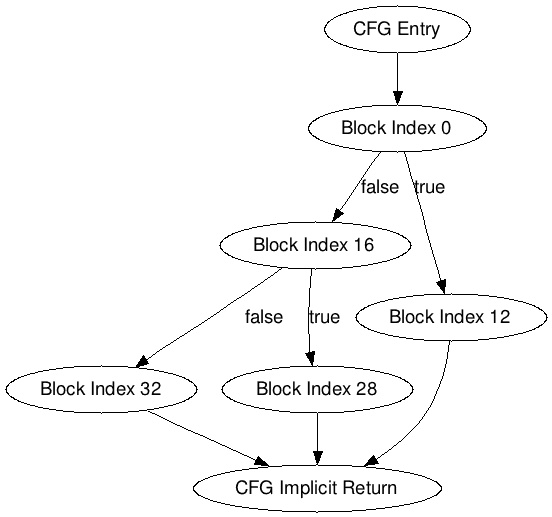• 深入Python解释器理解Python中的字节码
• 2017年12月24日
• 网络收集

Python是一门动态语言，在命令行工具下运行时，本质上执行了下面的步骤：

•     当第一次执行到一段代码时，这段代码会被编译（如，作为一个模块加载，或者直接执行）。根据操作系统的不同，这一步生成后缀名是pyc或者pyo的二进制文件。
•     解释器读取二进制文件，并依次执行指令（opcodes）。

Python解释器是基于栈的。要理解数据流向，我们需要知道每条指令的栈效应（如，操作码和参数）。

```import marshal
fd = open('path/to/my.pyc', 'rb')
fd.close()
```

code_object包含了一个CodeType对象，它代表被加载文件的整个模块。为了查看这个模块的类定义、方法等的所有嵌套编码对象（编码对象，原文为code object），我们需要递归地检查CodeType的常量池。就像下面的代码：

```import types
def inspect_code_object(co_obj, indent=''):
print indent, "%s(lineno:%d)" % (co_obj.co_name, co_obj.co_firstlineno)
for c in co_obj.co_consts:
if isinstance(c, types.CodeType):
inspect_code_object(c, indent + ' ')
inspect_code_object(code_object) # 从第一个对象开始
```

```class A:
def __init__(self):
pass
def __repr__(self):
return 'A()'
a = A()
print a
```

```<module>(lineno:2)
A(lineno:2)
__init__(lineno:3)
__repr__(lineno:5)
```

```co_obj = compile(python_source_code, '<string>', 'exec')
```

• ? 解释操作码的含义
• ? 提取任意参数

dis模块的disassemble函数展示了是如何做到的。对我们前面例子，它输出的结果是：

```2 0 LOAD_CONST 0 ('A')
6 LOAD_CONST 1 (<code object A at 0x42424242, file "<string>", line 2>)
9 MAKE_FUNCTION 0
12 CALL_FUNCTION 0
15 BUILD_CLASS
16 STORE_NAME 0 (A)
22 CALL_FUNCTION 0
25 STORE_NAME 1 (a)
31 PRINT_ITEM
32 PRINT_NEWLINE
36 RETURN_VALUE
```

•     行号（当它改变时）
•     指令的序号
•     当前指令的操作码
•     计算后的实际参数（圆括号内）

•     编译器优化
•     解释器优化（因此会导致加入额外的操作码）

```(1) a, b = 1, '2'
(2) a, b = 1, e
(3) a, b, c = 1, 2, e
(4) a, b, c, d = 1, 2, 3, e
```

```0 LOAD_CONST 5 ((1, '2'))
3 UNPACK_SEQUENCE 2
6 STORE_FAST 0 (a)
9 STORE_FAST 1 (b)
```

```12 LOAD_CONST 1 (1)
18 ROT_TWO
19 STORE_FAST 0 (a)
22 STORE_FAST 1 (b)
```

```25 LOAD_CONST 1 (1)
34 ROT_THREE
35 ROT_TWO
36 STORE_FAST 0 (a)
39 STORE_FAST 1 (b)
42 STORE_FAST 2 (c)
```

```45 LOAD_CONST 1 (1)
57 BUILD_TUPLE 4
60 UNPACK_SEQUENCE 4
63 STORE_FAST 0 (a)
66 STORE_FAST 1 (b)
69 STORE_FAST 2 (c)
72 STORE_FAST 3 (d)
```

Java中，invokeinterface，invokespecial和invokevirtual都是从静态类型语言中借鉴来的（invokespecial只被用来调用构造函数和父类AFAIK）。Invokestatic是自我描述的（不需要把接收方放在栈上），在Python中没有类似的概念（在解释器层面上，而不是装饰者）。简短的说，Python调用都能被转换成invokedynamic。

```func(arg1, arg2, keyword=SOME_VALUE, *unpack_list, **unpack_dict)
```

? 传递变量参数列表（_VAR）：CALL_FUNCTION_VAR, CALL_FUNCTION_VAR_KW
? 传递基于字典的关键字（_KW）：CALL_FUNCTION_KW, CALL_FUNCTION_VAR_KW

```0 LOAD_NAME 0 (func)
21 CALL_FUNCTION_VAR_KW 258
```

```na = arg & 0xff # num args
nk = (arg >> 8) & 0xff # num keywords
n_to_pop = na + 2 * nk + CALL_EXTRA_ARG_OFFSET[op]
```

CALL_EXTRA_ARG_OFFSET包含了一个偏移量，由调用操作码确定（对CALL_FUNCTION_VAR_KW来说，是2）。这里，在访问函数名称前，我们需要弹出6个元素。

JUMP_FORWARD：在字节码中跳转到一个相对位置。参数是跳过的字节数。
JUMP_IF_FALSE_OR_POP，JUMP_IF_TRUE_OR_POP，JUMP_ABSOLUTE，POP_JUMP_IF_FALSE，以及POP_JUMP_IF_TRUE：参数都是字节码中的绝对地址。

```def factorial(n):
if n <= 1:
return 1
elif n == 2:
return 2
return n * factorial(n - 1)
```

```module_co = compile(python_source, '', 'exec')
meth_co = module_co.co_consts
```

```3  0 LOAD_FAST  0 (n)
6 COMPARE_OP  1 (<=)
9 POP_JUMP_IF_FALSE 16  <<< control flow
15 RETURN_VALUE    <<< control flow
5 >> 16 LOAD_FAST  0 (n)
22 COMPARE_OP  2 (==)
25 POP_JUMP_IF_FALSE 32  <<< control flow
31 RETURN_VALUE    <<< control flow
7 >> 32 LOAD_FAST  0 (n)
44 BINARY_SUBTRACT
45 CALL_FUNCTION  1
48 BINARY_MULTIPLY
49 RETURN_VALUE    <<< control flow
```

POP_JUMP_IF_FALSE：跳转到绝对地址16和32；
RETURN_VALUE：从栈顶弹出一个元素，并返回。

```import opcode
RETURN_VALUE = 83
JUMP_FORWARD, JUMP_ABSOLUTE = 110, 113
FALSE_BRANCH_JUMPS = (111, 114) # JUMP_IF_FALSE_OR_POP, POP_JUMP_IF_FALSE
def find_blocks(meth_co):
blocks = {}
code = meth_co.co_code
finger_start_block = 0
i, length = 0, len(code)
while i < length:
op = ord(code[i])
i += 1
if op == RETURN_VALUE: # We force finishing the block after the return,
# dead code might still exist after though...
blocks[finger_start_block] = {
'length': i - finger_start_block - 1,
'exit': True
}
finger_start_block = i
elif op >= opcode.HAVE_ARGUMENT:
oparg = ord(code[i]) + (ord(code[i+1]) << 8)
i += 2
blocks[finger_start_block] = {
'length': i - finger_start_block
}
if op == JUMP_ABSOLUTE: # Only uncond absolute jump
blocks[finger_start_block]['conditions'] = {
'uncond': oparg
}
else:
false_index, true_index = (oparg, i) if op in FALSE_BRANCH_JUMPS else (i, oparg)
blocks[finger_start_block]['conditions'] = {
'true': true_index,
'false': false_index
}
finger_start_block = i
elif op in opcode.hasjrel:
# Essentially do the same...
pass
return blocks
```

```Block 0: {'length': 12, 'conditions': {'false': 16, 'true': 12}}
Block 12: {'length': 3, 'exit': True}
Block 16: {'length': 12, 'conditions': {'false': 32, 'true': 28}}
Block 28: {'length': 3, 'exit': True}
Block 32: {'length': 17, 'exit': True}
```

```Basic blocks
start_block_index :=
length := size of instructions
condition := true | false | uncond -> target_index
exit* := true
```

```def to_dot(blocks):
cache = {}
def get_node_id(idx, buf):
if idx not in cache:
cache[idx] = 'node_%d' % idx
buf.append('%s [label="Block Index %d"];' % (cache[idx], idx))
return cache[idx]
buffer = ['digraph CFG {']
buffer.append('entry [label="CFG Entry"]; ')
buffer.append('exit [label="CFG Implicit Return"]; ')
for block_idx in blocks:
node_id = get_node_id(block_idx, buffer)
if block_idx == 0:
buffer.append('entry -> %s;' % node_id)
if 'conditions' in blocks[block_idx]:
for cond_kind in blocks[block_idx]['conditions']:
target_id = get_node_id(blocks[block_idx]['conditions'][cond_kind], buffer)
buffer.append('%s -> %s [label="%s"];' % (node_id, target_id, cond_kind))
if 'exit' in blocks[block_idx]:
buffer.append('%s -> exit;' % node_id)
buffer.append('}')
return 'n'.join(buffer)
```